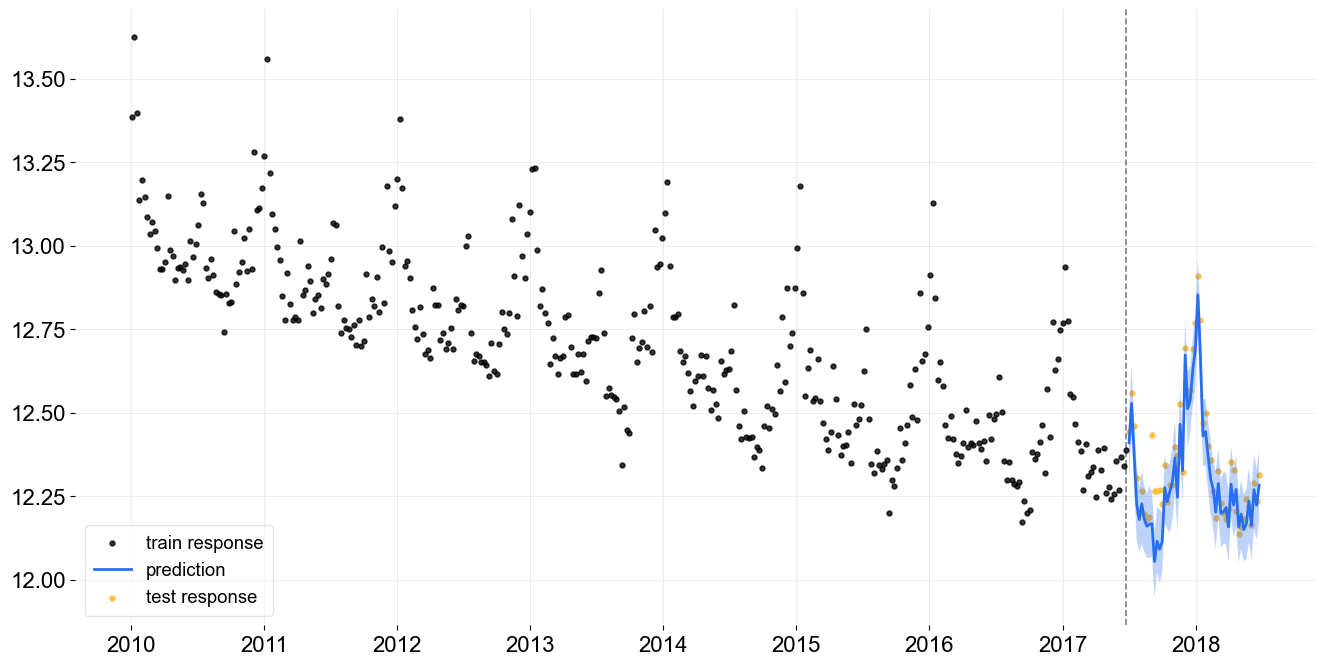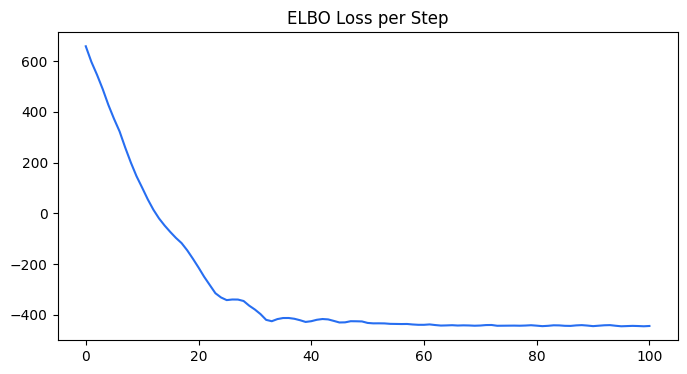# Using Pyro for Estimation¶

Note

Currently we are still experimenting with Pyro and support Pyro only in LGT and KTR models.

Pyro is a flexible, scalable deep probabilistic programming library built on PyTorch. Pyro was originally developed at Uber AI and is now actively maintained by community contributors, including a dedicated team at the Broad Institute.

:

%matplotlib inline

import pandas as pd
import numpy as np
import matplotlib.pyplot as plt

import orbit
from orbit.models import LGT
from orbit.diagnostics.plot import plot_predicted_data
from orbit.diagnostics.plot import plot_predicted_components

from orbit.constants.palette import OrbitPalette

:

print(orbit.__version__)

1.1.4

:

df = load_iclaims()

:

test_size=52
train_df=df[:-test_size]
test_df=df[-test_size:]


## VI Fit and Predict¶

Although Pyro provides a variety of ways to optimize/sample posteriors. Currently, we only support Stochastic Variational Inference (SVI). For details, please refer to this doc.

To use SVI for LGT, specify estimator as pyro-svi.

:

lgt_vi = LGT(
response_col='claims',
date_col='week',
seasonality=52,
seed=8888,
estimator='pyro-svi',
num_steps=101,
num_sample=300,
# trigger message per 50 steps
message=50,
learning_rate=0.1,
)

:

%%time
lgt_vi.fit(df=train_df)

2023-01-20 20:33:10 - orbit - INFO - Using SVI (Pyro) with steps: 101, samples: 300, learning rate: 0.1, learning_rate_total_decay: 1.0 and particles: 100.
2023-01-20 20:33:10 - orbit - INFO - step    0 loss = 658.91, scale = 0.11635
INFO:orbit:step    0 loss = 658.91, scale = 0.11635
2023-01-20 20:33:13 - orbit - INFO - step   50 loss = -432, scale = 0.48623
INFO:orbit:step   50 loss = -432, scale = 0.48623
2023-01-20 20:33:16 - orbit - INFO - step  100 loss = -444.07, scale = 0.34976
INFO:orbit:step  100 loss = -444.07, scale = 0.34976

CPU times: user 6.08 s, sys: 571 ms, total: 6.65 s
Wall time: 6.41 s

:

<orbit.forecaster.svi.SVIForecaster at 0x2a67c3a30>

:

predicted_df = lgt_vi.predict(df=test_df)

:

_ = plot_predicted_data(training_actual_df=train_df, predicted_df=predicted_df,
date_col=lgt_vi.date_col, actual_col=lgt_vi.response_col,
test_actual_df=test_df)We can also extract the ELBO loss from the training metrics.

:

loss_elbo = lgt_vi.get_training_metrics()['loss_elbo']

:

steps = np.arange(len(loss_elbo))
plt.subplots(1, 1, figsize=(8, 4))
plt.plot(steps, loss_elbo, color=OrbitPalette.BLUE.value)
plt.title('ELBO Loss per Step')

:

Text(0.5, 1.0, 'ELBO Loss per Step')